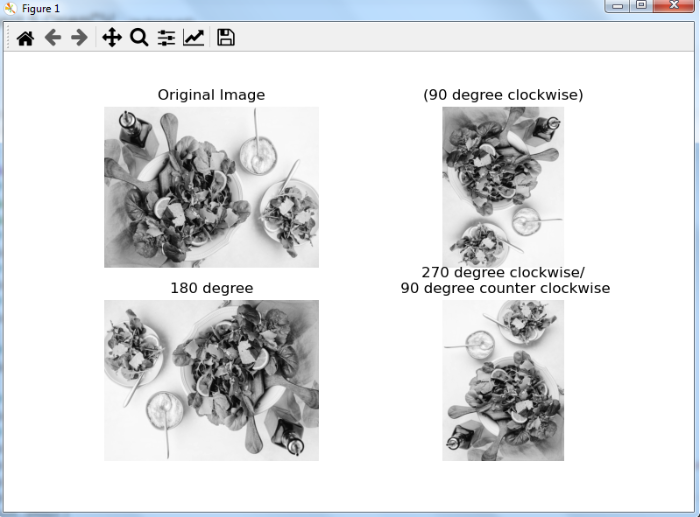# How to rotate an image in OpenCV Python?

OpenCV provides us the function cv.rotate() to rotate an image (numpy array) in multiples of 90 degrees. This function rotates an image in three possible ways: 90, 180, and 270 degrees clockwise. We use the following syntax −

## Syntax


cv2.rotate(img, rotateCode)


rotateCode is a rotate flag specifying how to rotate the array. The three rotate flags are as below −

• cv2.ROTATE_90_CLOCKWISE

• cv2.ROTATE_180

• cv2.ROTATE_90_COUNTERCLOCKWISE

## Steps

To rotate an input image, you could follow the steps given below −

• Import the required libraries OpenCV and matplotlib. Make sure you have already installed them.

• Read the input image using cv2.imread() method. Specify the full path of the image.

• Rotate the input image using cv2.rotate() function. Pass the desired rotateCode to the function as a parameter. We can pass cv2.ROTATE_180, cv2.ROTATE_90_CLOCKWISE or cv2.ROTATE_90_COUNTERCLOCKWISE as a parameter.

• Display the rotated image.

Let's see the examples to rotate an input image.

## Input Image

We will use the following image as an input file in the examples below −## Example

In this example, we rotate the input image by 180 degrees clockwise.

# import required libraries
import cv2

# rotate the image by 180 degree clockwise
img_cw_180 = cv2.rotate(img, cv2.ROTATE_180)

# display the rotated image
cv2.imshow("Image rotated by 180 degree", img_cw_180)
cv2.waitKey(0)
cv2.destroyAllWindows()


## Output

When you execute the above program, it will produce the following output window −Notice that the input image is rotated 180 degrees clockwise.

Let's see other rotations available in OpenCV.

## Example

In this example, we show how to rotate the input image by 90, 180, and 270 degrees.

# import required libraries
import cv2
import matplotlib.pyplot as plt

# convert the image to grayscale
img = cv2.cvtColor(img, cv2.COLOR_BGR2GRAY)

# rotate the image by 90 degree clockwise
img_cw_90 = cv2.rotate(img, cv2.ROTATE_90_CLOCKWISE)

# rotate the image by 270 degree clockwise or 90 degree counterclockwise
img_ccw_90 = cv2.rotate(img, cv2.ROTATE_90_COUNTERCLOCKWISE)

# rotate the image by 180 degree clockwise
img_cw_180 = cv2.rotate(img, cv2.ROTATE_180)

# display all the images
plt.subplot(221), plt.imshow(img, 'gray'), plt.title('Original
Image'), plt.axis('off')
plt.subplot(222), plt.imshow(img_cw_90,'gray'), plt.title('(90
degree clockwise)'), plt.axis('off')
plt.subplot(223), plt.imshow(img_cw_180, 'gray'),
plt.title('180 degree'), plt.axis('off')
plt.subplot(224), plt.imshow(img_ccw_90, 'gray'),
plt.title('270 degree clockwise/\n 90 degree counter
clockwise'), plt.axis('off')
plt.show()


## Output

The above program, on execution, will produce the following output window −